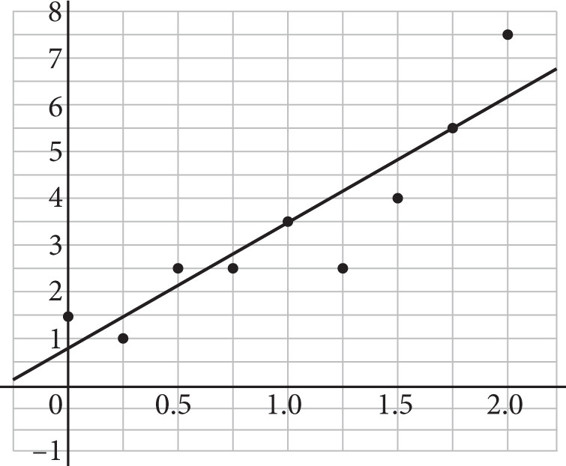# SAT Math Multiple Choice Question 435: Answer and Explanation

### Test Information

Question: 435

15. Use the data in the scatterplot and the line of best fit shown to answer the following question.According to the graph, the data has been modeled using a line of best fit. Another researcher thinks that an exponential model may be a better fit. The table below shows the researcher's results after using a graphing calculator to perform a linear regression and an exponential regression on the data.

 LinReg ExpReg y = ax + b y = a × bx a = 2.7 a = 1.251327 b = .68888889 b = 2.299749 r2 = .81876039 r2 = .84304281 r = .9048538 r = .9181736

Which of the following best explains which regression model is a better fit and why?

• A. A linear model is a much better fit because its value of a is considerably higher.
• B. A linear model is a slightly better fit because its value of r is slightly smaller.
• C. An exponential model is a much better fit because its value of a is much closer to 1.
• D. An exponential model is a slightly better fit because its value of r is slightly closer to 1.